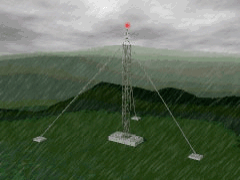Ch 4. Fundamental Laws (Integral Anal.) Multimedia Engineering Fluids ConservationMass LinearMomentum Moment ofMomentum ConservationEnergy
 Chapter 1. Basics 2. Fluid Statics 3. Kinematics 4. Laws (Integral) 5. Laws (Diff.) 6. Modeling/Similitude 7. Inviscid 8. Viscous 9. External Flow 10. Open-Channel Appendix Basic Math Units Basic Equations Water/Air Tables Sections Search eBooks Dynamics Fluids Math Mechanics Statics Thermodynamics Author(s): Chean Chin Ngo Kurt Gramoll ©Kurt GramollFLUID MECHANICS - EXAMPLE QuestionsProblem Diagram Rain falls at a uniform rate of 2 cm/hr over a 1 km x 1 km field, the water infiltrates into the soil at a rate of 1 cm/hr. At the downstream end of the field, the small channel carries a flow of 0.01 m3/s. If all rates remain constant, determine if ponding occurs. Solution Rain falls at: i = 2 cm/hr = 0.02 m/hr over an area of A = 1 km2 = 1,000,000 m2 Infiltration rate: 1 cm/hr = 0.01 m/hr A small channel causes runoff = 0.01 m3/s = 36 m3/hr For ponding, rainfall must exceed infiltration plus runoff (conservation of mass for a constant density fluid)      rainfall volume      = (rainfall intensity)(area) = iA      = (0.02)(1,000,000) = 20,000 m3/hr      infiltration volume + runoff volume      = (0.01)(1,000,000) + 36      = 10,036 m3/hr Since rainfall volume > (infiltration volume + runoff volume), ponding will occur at a rate of (20,000 - 10,036) = 9,964 m3/hr Note: system is not at steady state. For it to be at steady state, the outflow would need to increase to 10,000 m3/hr, at which point there would be zero ponding.

Practice Homework and Test problems now available in the 'Eng Fluids' mobile app
Includes over 250 problems with complete detailed solutions.
Available now at the Google Play Store and Apple App Store.